# Join Data Frames with the R dplyr Package (9 Examples)

In this R programming tutorial, I will show you how to merge data with the join functions of the dplyr package. More precisely, I’m going to explain the following functions:

First I will explain the basic concepts of the functions and their differences (including simple examples). Afterwards, I will show some more complex examples:

So without further ado, let’s get started!

## Simple Example Data

Before we can start with the introductory examples, we need to create some data in R:

```data1 <- data.frame(ID = 1:2, # Create first example data frame X1 = c("a1", "a2"), stringsAsFactors = FALSE) data2 <- data.frame(ID = 2:3, # Create second example data frame X2 = c("b1", "b2"), stringsAsFactors = FALSE)```

Figure 1 illustrates how our two data frames look like and how we can merge them based on the different join functions of the dplyr package.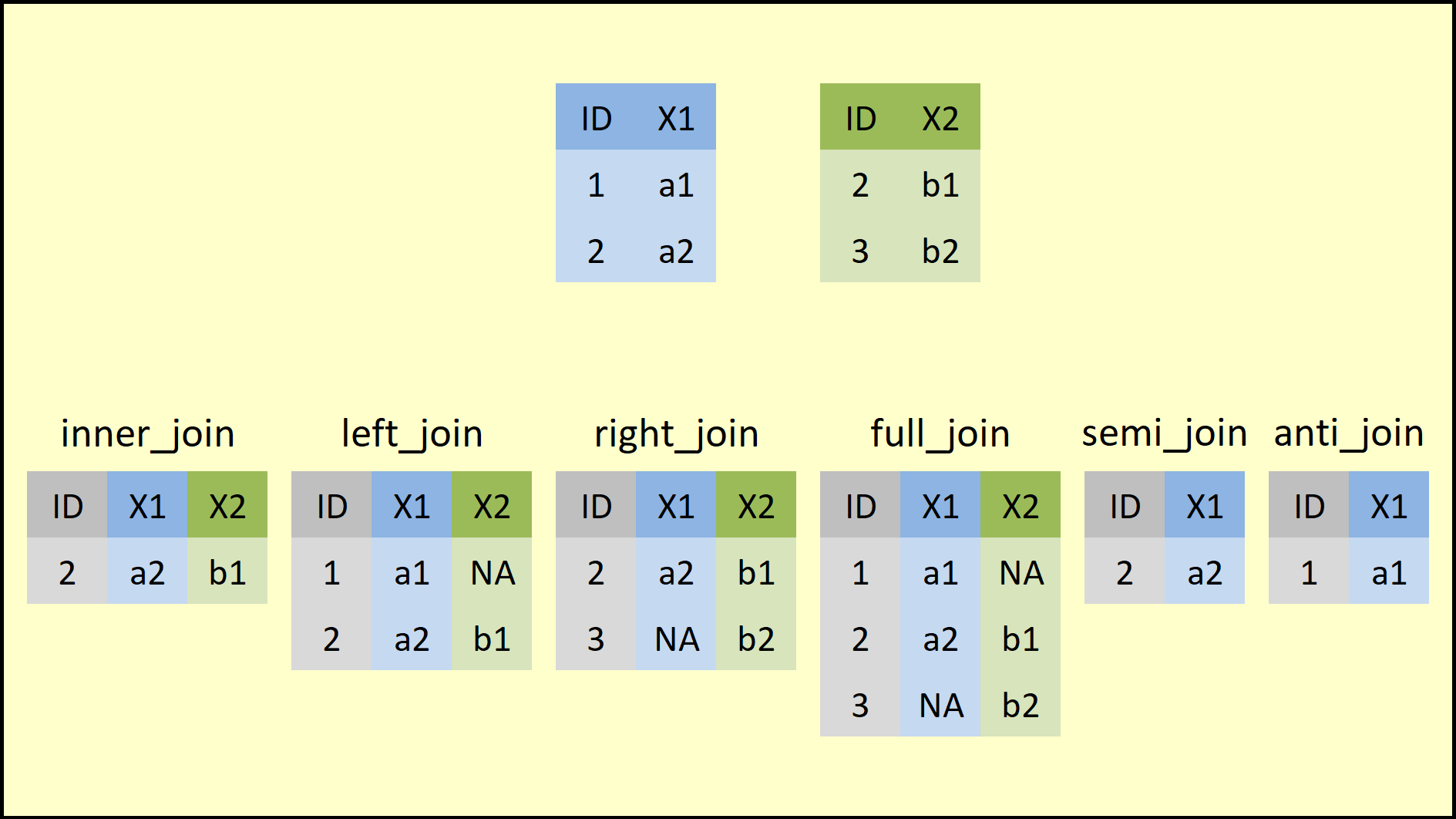Figure 1: Overview of the dplyr Join Functions.

On the top of Figure 1 you can see the structure of our example data frames. Both data frames contain two columns: The ID and one variable. Note that both data frames have the ID No. 2 in common.

On the bottom row of Figure 1 you can see how each of the join functions merges our two example data frames. However, I’m going to show you that in more detail in the following examples…

By the way: I have also recorded a video, where I’m explaining the following examples. If you prefer to learn based on a video, you might check out the following video of my YouTube channel:

Please accept YouTube cookies to play this video. By accepting you will be accessing content from YouTube, a service provided by an external third party.If you accept this notice, your choice will be saved and the page will refresh.

## Example 1: inner_join dplyr R Function

Before we can apply dplyr functions, we need to install and load the dplyr package into RStudio:

```install.packages("dplyr") # Install dplyr package library("dplyr") # Load dplyr package```

In this first example, I’m going to apply the inner_join function to our example data.

In order to merge our data based on inner_join, we simply have to specify the names of our two data frames (i.e. data1 and data2) and the column based on which we want to merge (i.e. the column ID):

`inner_join(data1, data2, by = "ID") # Apply inner_join dplyr function`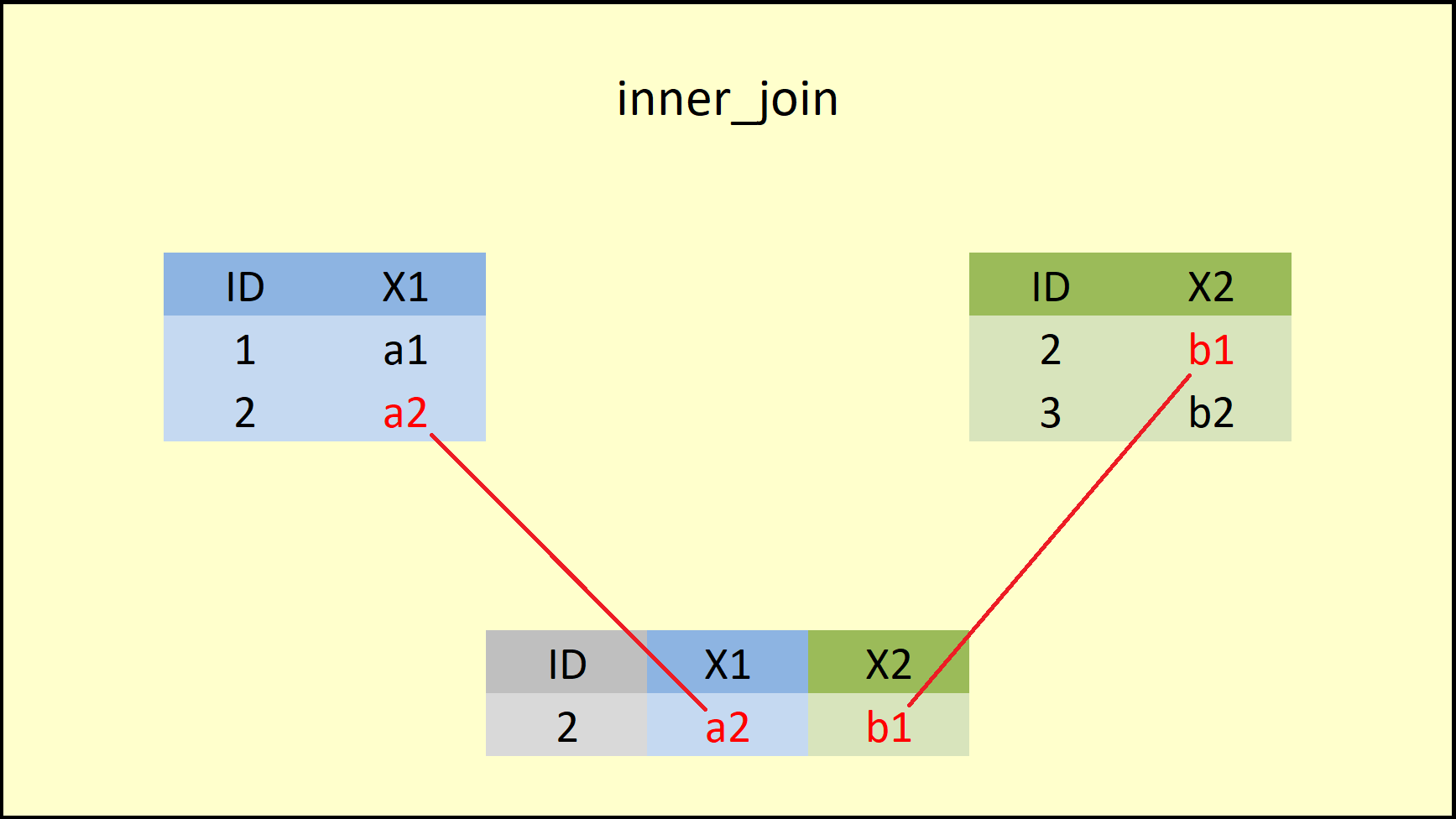Figure 2: dplyr inner_join Function.

Figure 2 illustrates the output of the inner join that we have just performed. As you can see, the inner_join function merges the variables of both data frames, but retains only rows with a shared ID (i.e. ID No. 2).

More precisely, this is what the R documentation is saying:So what is the difference to other dplyr join functions? Let’s move on to the next command.

## Example 2: left_join dplyr R Function

The left_join function can be applied as follows:

`left_join(data1, data2, by = "ID") # Apply left_join dplyr function`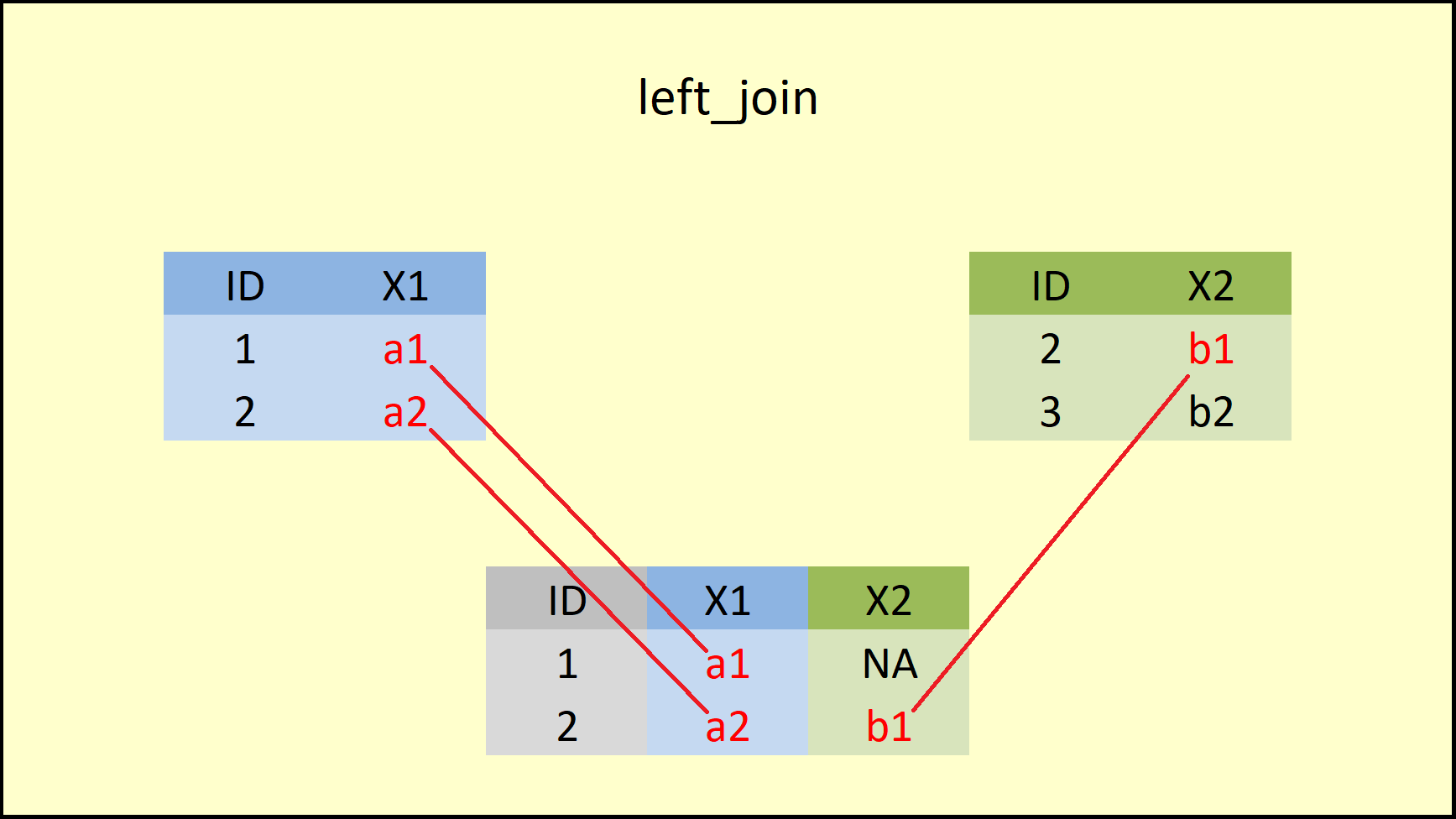Figure 3: dplyr left_join Function.

The difference to the inner_join function is that left_join retains all rows of the data table, which is inserted first into the function (i.e. the X-data). Have a look at the R documentation for a precise definition: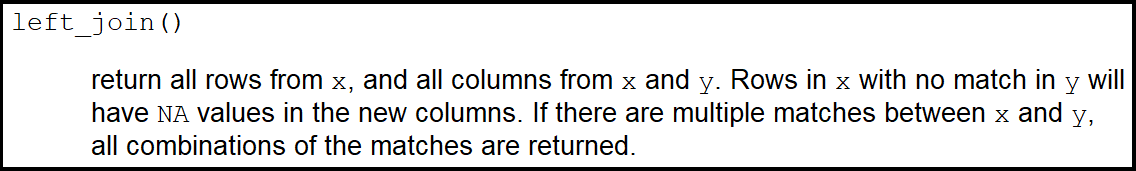## Example 3: right_join dplyr R Function

Right join is the reversed brother of left join:

`right_join(data1, data2, by = "ID") # Apply right_join dplyr function`Figure 4: dplyr right_join Function.

Figure 4 shows that the right_join function retains all rows of the data on the right side (i.e. the Y-data). If you compare left join vs. right join, you can see that both functions are keeping the rows of the opposite data.

This behavior is also documented in the definition of right_join below:So what if we want to keep all rows of our data tables? That’s exactly what I’m going to show you next!

## Example 4: full_join dplyr R Function

A full outer join retains the most data of all the join functions. Let’s have a look:

`full_join(data1, data2, by = "ID") # Apply full_join dplyr function`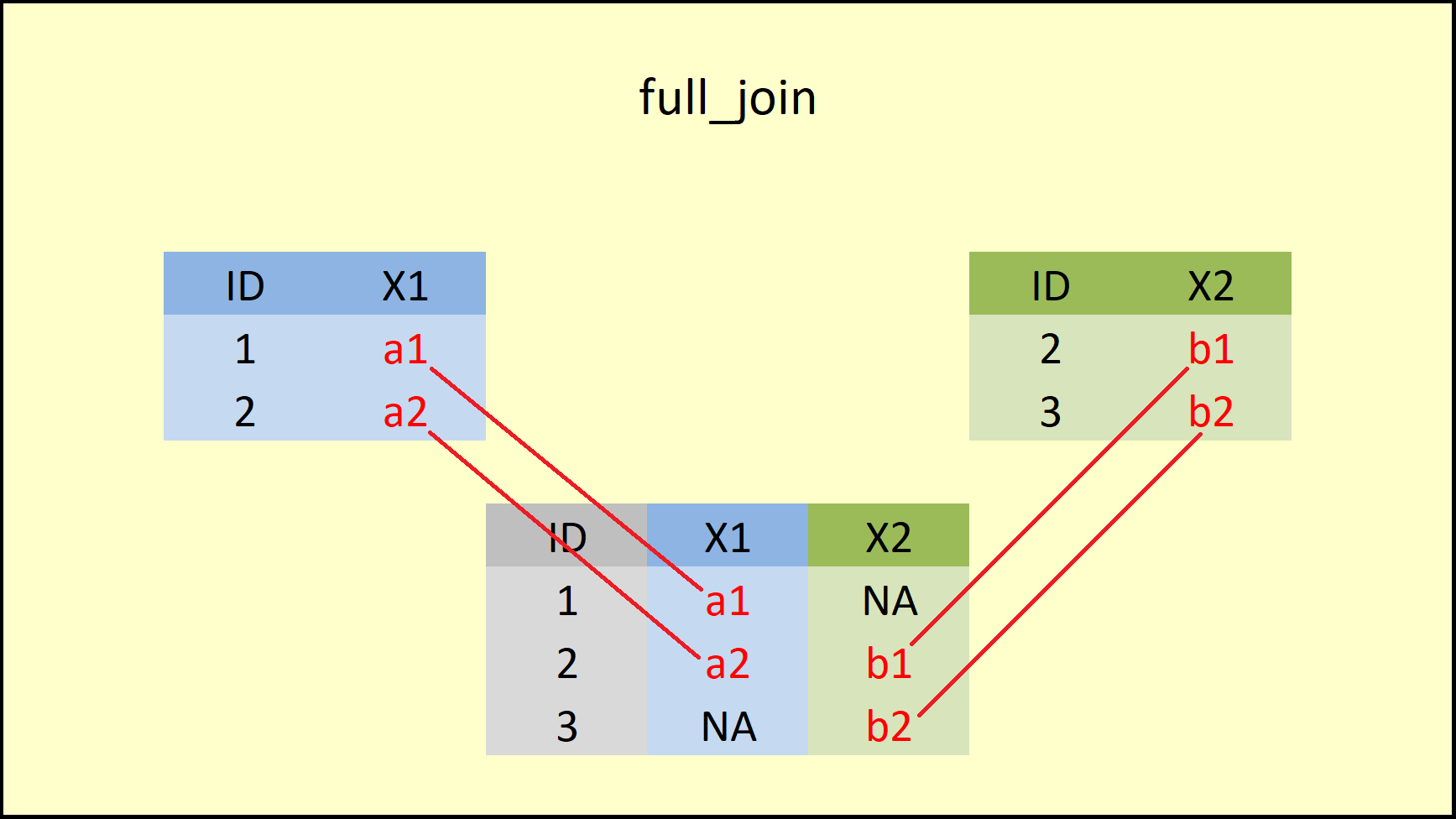Figure 5: dplyr full_join Function.

As Figure 5 illustrates, the full_join functions retains all rows of both input data sets and inserts NA when an ID is missing in one of the data frames.

You can find the help documentation of full_join below: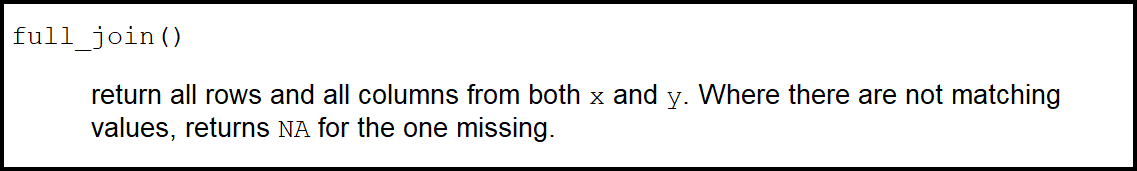## Example 5: semi_join dplyr R Function

The four previous join functions (i.e. inner_join, left_join, right_join, and full_join) are so called mutating joins. Mutating joins combine variables from the two data sources.

The next two join functions (i.e. semi_join and anti_join) are so called filtering joins. Filtering joins keep cases from the left data table (i.e. the X-data) and use the right data (i.e. the Y-data) as filter.

Let’s have a look at semi join first:

`semi_join(data1, data2, by = "ID") # Apply semi_join dplyr function`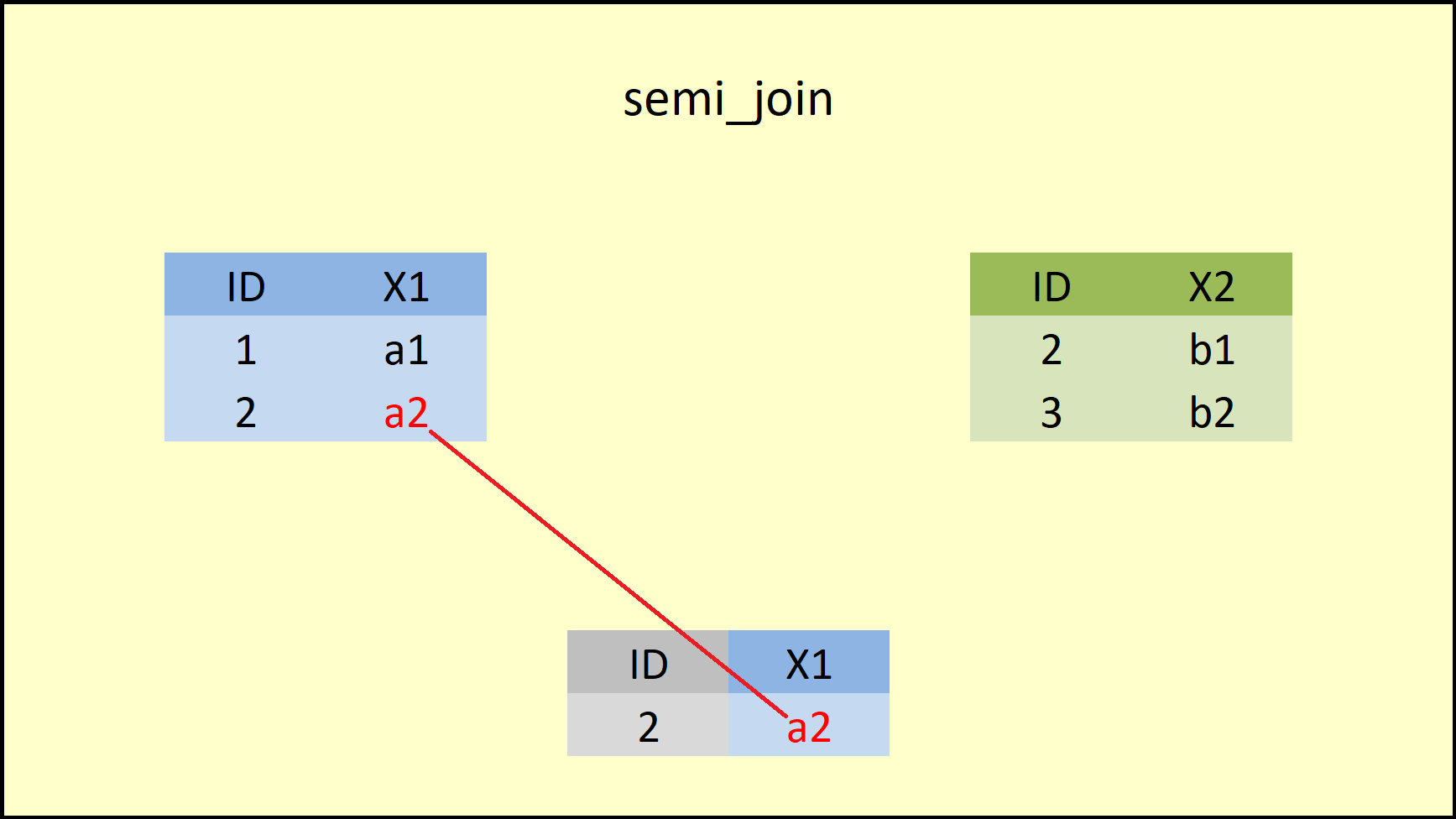Figure 6: dplyr semi_join Function.

Figure 6 illustrates what is happening here: The semi_join function retains only rows that both data frames have in common AND only columns of the left-hand data frame. You can find a precise definition of semi join below: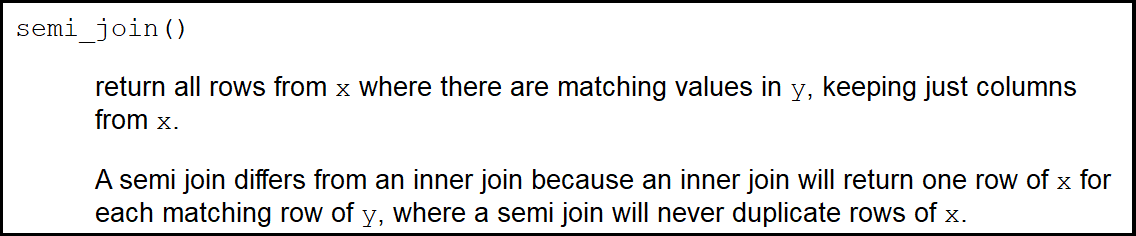## Example 6: anti_join dplyr R Function

Anti join does the opposite of semi join:

`anti_join(data1, data2, by = "ID") # Apply anti_join dplyr function`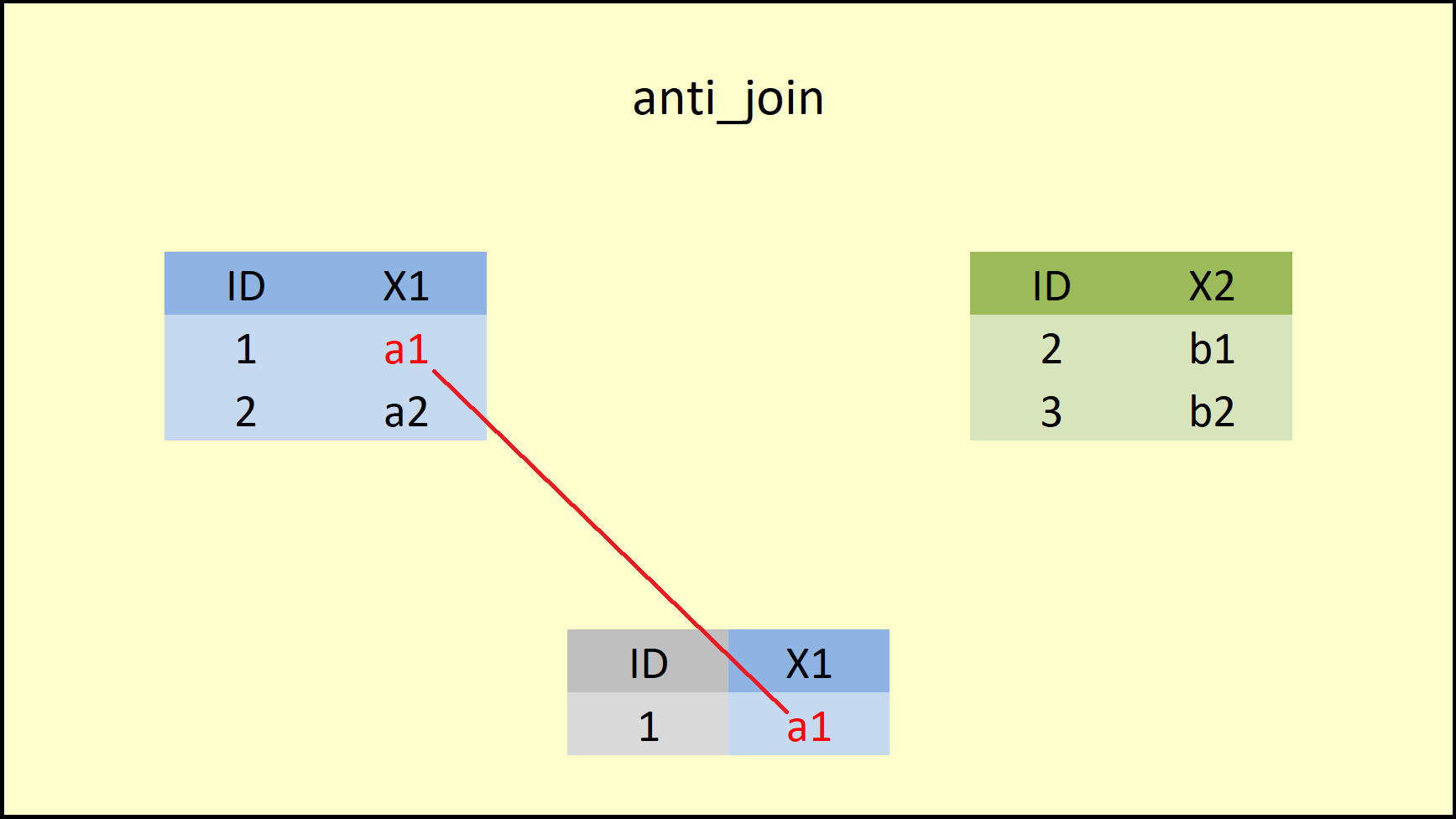Figure 7: dplyr anti_join Function.

As you can see, the anti_join functions keeps only rows that are non-existent in the right-hand data AND keeps only columns of the left-hand data. The R help documentation of anti join is shown below:At this point you have learned the basic principles of the six dplyr join functions. However, in practice the data is of cause much more complex than in the previous examples. In the remaining tutorial, I will therefore apply the join functions in more complex data situations.

Let’s dive in!

## Example 7: Join Multiple Data Frames

To make the remaining examples a bit more complex, I’m going to create a third data frame:

```data3 <- data.frame(ID = c(2, 4), # Create third example data frame X2 = c("c1", "c2"), X3 = c("d1", "d2"), stringsAsFactors = FALSE) data3 # Print data to RStudio console # ID X2 X3 # 2 c1 d1 # 4 c2 d2```

The third data frame data3 also contains an ID column as well as the variables X2 and X3. Note that the variable X2 also exists in data2.

In this example, I’ll explain how to merge multiple data sources into a single data set. For the following examples, I’m using the full_join function, but we could use every other join function the same way:

```full_join(data1, data2, by = "ID") %>% # Full outer join of multiple data frames full_join(., data3, by = "ID") # ID X1 X2.x X2.y X3 # 1 a1 <NA> <NA> <NA> # 2 a2 b1 c1 d1 # 3 <NA> b2 <NA> <NA> # 4 <NA> <NA> c2 d2```

As you can see based on the previous code and the RStudio console output: We first merged data1 and data2 and then, in the second line of code, we added data3.

Note that X2 was duplicated, since it exists in data2 and data3 simultaneously. In the next example, I’ll show you how you might deal with that.

## Example 8: Join by Multiple Columns

As you have seen in Example 7, data2 and data3 share several variables (i.e. ID and X2). If we want to combine two data frames based on multiple columns, we can select several joining variables for the by option simultaneously:

```full_join(data2, data3, by = c("ID", "X2")) # Join by multiple columns # ID X2 X3 # 2 b1 <NA> # 3 b2 <NA> # 2 c1 d1 # 4 c2 d2```

Note: The row of ID No. 2 was replicated, since the row with this ID contained different values in data2 and data3.

## Example 9: Join Data & Delete ID

In the last example, I want to show you a simple trick, which can be helpful in practice. Often you won’t need the ID, based on which the data frames where joined, anymore. In order to get rid of the ID efficiently, you can simply use the following code:

```inner_join(data1, data2, by = "ID") %>% # Automatically delete ID select(- ID) # X1 X2 # a2 b1```

That’s it, clean and simple!

In this R tutorial, I’ve shown you everything I know about the dplyr join functions.

Now I would like to hear from you!

Which is your favorite join function? Do you prefer to keep all data with a full outer join or do you use a filter join more often?

Subscribe to the Statistics Globe Newsletter

•Narasimhan Kothandaraman
April 10, 2019 2:08 am

Hi Joachim,
Your representation of the join function is the best I have ever seen. It’s so good for people like me who are beginners in R programming.

Hope to see more on :

1) reading excel files in R

2) saving excel files in R

3) collating multiple excel files into one single excel file with multiple sheets
and
4) creating summary tables with p-values for categorical, continuous and non-normalised data that are
ready to publish as subject characteristics in cohort studies.

Many thanks,
Nara

• Hey Nara, thank you so much for the awesome comment. Didn’t expect such a nice feedback! Based on your request, I have just published a tutorial on how to export data from R to Excel. You can find the tutorial here: https://statisticsglobe.com/write-xlsx-xls-export-data-from-r-to-excel-file I also put your other wishes on my short-term to do list. You can expect more tutorials soon. Thanks, Joachim

•Alexis
October 16, 2019 11:32 pm

Hello Joachim,

Thank you very much for the join data frame explanation, it was clear and I learned from it. Graphically it was easy to understand the concepts. Hope the best for you.

Kind regards.

• Thank you very much Alexis. It’s very nice to get such a positive feedback!

Regards,

Joachim

•Ioannis
February 25, 2020 8:48 pm

Great job, clear and very thorough description. I am teaching a series of courses in R and I will recommend your post to my students to check out when they want to learn more about join with dplyr!

• This is very nice to hear Ioannis! Thanks for letting your students know about my site 🙂

•Andrew Ward
May 13, 2020 3:11 pm

I was going around in circles with this join function on a course where they were using much more complex databases. I understood significantly better now. Thanks for this!

• This is great to hear Andrew! Glad I was able to help 🙂

Regards,

Joachim

•Jon Crowe
September 14, 2020 2:45 pm

Hi Joachim, thanks for these really clear visual examples of join functions – just what I was looking for! I’ve bookmarked your site and I’m sure I’ll be back as my R learning continues.

Jon

• Hi Jon,

Thanks a lot for the awesome feedback! Glad to hear you like my content 🙂

Regards,

Joachim

•@katazen
January 3, 2021 6:04 pm

Great explanation…as usual. Let me pointing out a typo without stealing credit from your article:”Note that X2 was duplicated, since it exists in data1 and data2 simultaneously.” I think you did mean “…in data2 and data3”. I’m going to your next reading!

• Hey Katazen,

Thanks a lot for the very kind words. I’m happy to hear that you enjoy reading my content! 🙂

Also, thanks a lot for mentioning the typo. It’s great that you were reading the article so carefully. I just fixed the typo.

Thanks

Joachim

•Ujjwal
April 28, 2021 4:30 am

Hi your representation on joints are good. Please make video on advance joints like using function suffix.

• Hey Ujjwal,

Thank you for the comment, glad you like it! Could you explain in some more detail what you mean with “function suffix”?

Regards

Joachim

•BRUNO
September 1, 2021 5:46 am

Hello Joachim. Congrats for The amazing work. A silly question: The Join family only van vê used for data frames with same dimesions? If so, what should I do? Thanks.

• Hey Bruno,

Thank you for the kind words!

The join family can also be used for data frames with different dimensions. Depending on the type of join you use, the rows with IDs that are only contained in one of the data frames will be removed.

Regards

Joachim

•D'Amour
September 15, 2021 1:47 pm

I am always wondering why Joachim does not publish an R book for beginners and I can testify that he will be the world best seller within a short moment! I appreciate the way you articulate things with simple and understandable approach. Whenever I am stuck over flow I eventually get a way back just from here.
GOD Bless you richly #Joachim!